# Lecture 15 (4/29/2022)¶

Announcements

• Pset 4 will be released today, due next Friday 5/6

Last time we covered:

• Evaluating regression: $$R^2$$, out-of-sample prediction, parameter interpretation

Today’s agenda:

• Polynomial regression, multiple regression

import numpy as np
import pandas as pd
import matplotlib.pyplot as plt
import seaborn as sns

# Read in and prepare the data we were using last time
mpg_clean = mpg.dropna().reset_index(drop = True)

mpg_clean

mpg cylinders displacement horsepower weight acceleration model_year origin name
0 18.0 8 307.0 130.0 3504 12.0 70 usa chevrolet chevelle malibu
1 15.0 8 350.0 165.0 3693 11.5 70 usa buick skylark 320
2 18.0 8 318.0 150.0 3436 11.0 70 usa plymouth satellite
3 16.0 8 304.0 150.0 3433 12.0 70 usa amc rebel sst
4 17.0 8 302.0 140.0 3449 10.5 70 usa ford torino
... ... ... ... ... ... ... ... ... ...
387 27.0 4 140.0 86.0 2790 15.6 82 usa ford mustang gl
388 44.0 4 97.0 52.0 2130 24.6 82 europe vw pickup
389 32.0 4 135.0 84.0 2295 11.6 82 usa dodge rampage
390 28.0 4 120.0 79.0 2625 18.6 82 usa ford ranger
391 31.0 4 119.0 82.0 2720 19.4 82 usa chevy s-10

392 rows × 9 columns

# Review: Evaluating linear regression¶

In last lecture, we talked about three ways of checking that your regression fit the data well.

1. $$R^2$$ coefficient of determination

2. Out of sample prediction accuracy

3. High confidence (and useful) parameter estimates

Let’s start by running through each of these in a little more detail since we didn’t get much time to discuss them.

## $$R^2$$, the coefficient of determination¶$$R^2 = 1 - \dfrac{RSS}{TSS}$$

$$RSS = \sum_{i=1}^{n}{(y_i - \hat{y_i})}^2$$

$$TSS = \sum_{i=1}^{n}{(y_i - \bar{y})}^2$$

$$R^2$$ ranges between 0 and 1 and can be thought of as the percentage of variance in $$y$$ that our model explains.

To understand how it works, remember that RSS is 0 when the regression perfectly predicts our data and RSS is equal to TSS when we just guess $$\bar{y}$$ for every data point $$y_i$$ (worst case for our regression).

# The scikit-learn LinearRegression class surfaces a function called score that computes R^2
from sklearn.linear_model import LinearRegression

# Format values
x_vals = np.array(mpg_clean['weight']).reshape(len(mpg_clean['weight']), 1)
y_vals = np.array(mpg_clean['horsepower'])

# Fit regression
mod = LinearRegression().fit(X = x_vals, y = y_vals)

rsq_mod = mod.score(X = x_vals, y = y_vals) # R^2 value
rsq_mod

0.74742549968982


Last time, we showed how to calculate the $$R^2$$ value by hand using the LinearRegression predict function.

If you’re feeling hazy on $$R^2$$, I recommend going back to the code from that lecture and going through the part where we calculate $$R^2$$.

## Out of sample prediction¶Motivation

If our model is the right fit to our data, it should predict other data from the same underlying distribution or generative process pretty well.

How to check this

There are a lot of ways to test out of sample data which we’ll get into in more detail on Monday, but the high-level approach is almost always:

1. Randomly select a subset of your original data (20-25%) and set it aside as test data. The remaining data is your training data.

2. Fit your model to the training data only.

3. See how well your fitted model predicts the test data. Compare it to the predictions on the training data with something like Mean Squared Error (MSE).

4. Often, repeat steps 1-3 in various ways (more on that later).

Comparing train and test performance

Step 3 above is critical. One common approach is to use Mean Squared Error (MSE):

$$MSE = \dfrac{1}{n - 2} \sum_{i=1}^{n}{(y_i - \hat{y_i})}^2 = \dfrac{1}{n - 2} \sum_{i=1}^{n}{\epsilon_i}^2$$

This tells you, on average, how close your model was to the true value across all the data points (the $$n-2$$ is specific to linear regression where we have two parameters, $$\beta_0$$ and $$\beta_1$$, so $$n-2$$ is our degrees of freedom).

from sklearn.model_selection import train_test_split # Use the sklearn train_test_split to make this easy
from sklearn.metrics import mean_squared_error # Use the sklearn mean_squared_error for quick MSE calculation

# Randomly sample 25% of our data points to be test data
xtrain, xtest, ytrain, ytest = train_test_split(x_vals,
y_vals,
test_size = 0.25,
#                                                 random_state = 500
)

# Fit the model on the training data
mod_tr = LinearRegression().fit(X = xtrain, y = ytrain)

# Generate model predictions for the test data
mod_preds_test = mod_tr.predict(X = xtest)

# Compare MSE for the model predictions on train and test data
mod_preds_train = mod_tr.predict(X = xtrain)

mse_train = mean_squared_error(y_true = ytrain, y_pred = mod_preds_train)
mse_train # Note this divides by n rather than n-2 but that's not super important for our purposes

mse_test = mean_squared_error(y_true = ytest, y_pred = mod_preds_test)
mse_test

print("Training MSE: {} \nTest MSE: {}".format(mse_train, mse_test))

Training MSE: 299.61077052468573
Test MSE: 596.445782974599


Just for fun, try running the code above several times and look at how different the values are.

More on this next week…

## Parameter estimates¶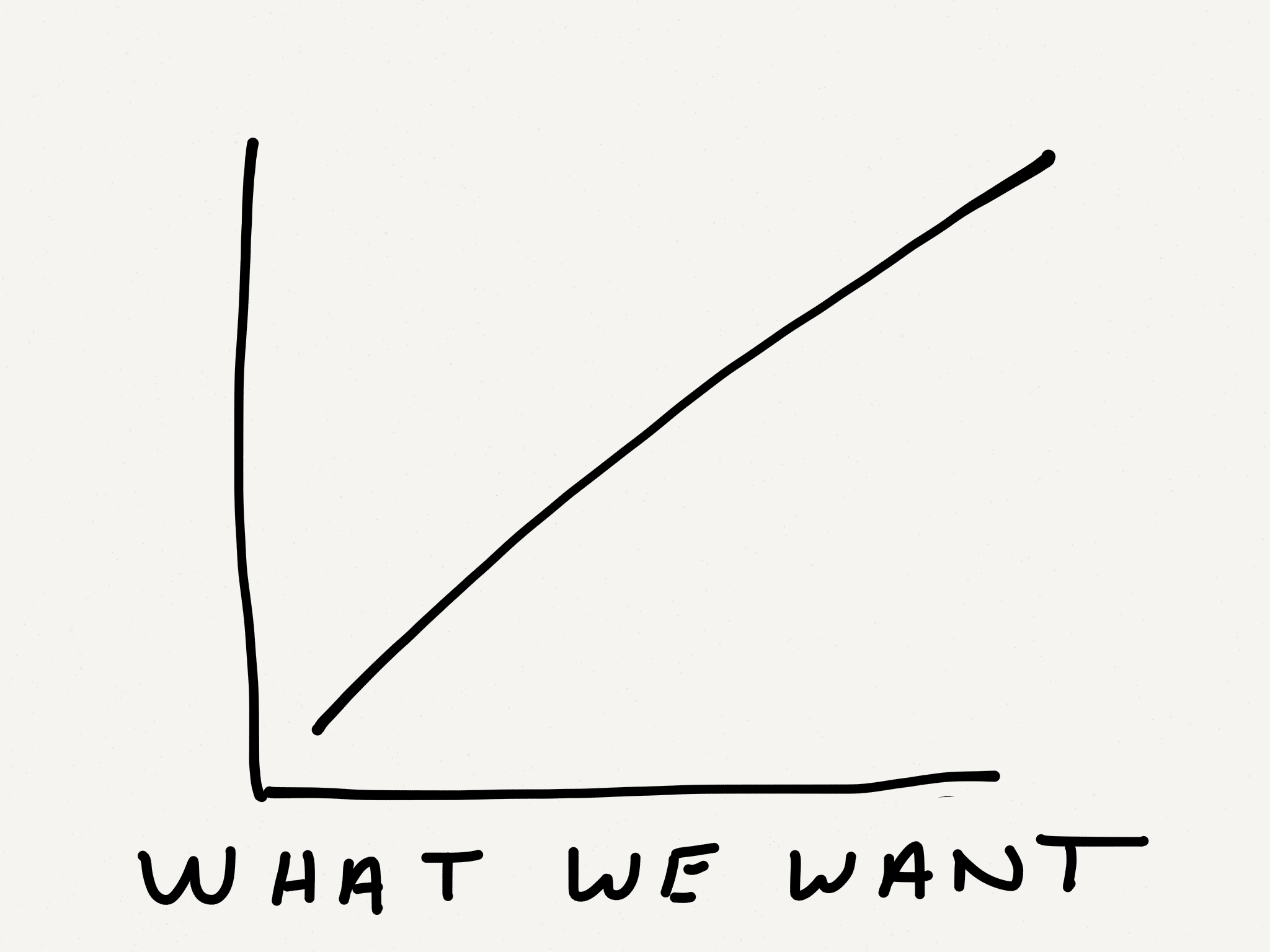The criteria above are mostly concerned with whether we’re doing a good job predicting our $$y$$ values with this model.

In many cases, part of what we’re concerned with isn’t just how well we predict our data, but what kind of relationship our model estimates between $$x$$ and $$y$$.

• How large or small is the slope?

• How confident are we in the estimate?

To assess this, we typically compute confidence bounds on the parameter estimates (95% confidence interval or standard error) and compare them to a null value of 0 using $$t$$ tests.

Linear regression parameter estimates are most useful when they are high confidence and significantly different from 0.

The sklearn LinearRegression class doesn’t include functions for this sort of analysis, but other tools like the statsmodels regression class do.

import statsmodels.formula.api as smf

# Fit the model
results = smf.ols('horsepower ~ weight', data = mpg_clean).fit()

# View the results
results.summary()

Dep. Variable: R-squared: horsepower 0.747 OLS 0.747 Least Squares 1154. Tue, 14 Jun 2022 1.36e-118 18:22:25 -1717.0 392 3438. 390 3446. 1 nonrobust
coef std err t P>|t| [0.025 0.975] -12.1835 3.570 -3.412 0.001 -19.203 -5.164 0.0392 0.001 33.972 0.000 0.037 0.041
 Omnibus: Durbin-Watson: 83.255 1.014 0 312.937 0.892 1.11e-68 6.997 11300

Notes:
 Standard Errors assume that the covariance matrix of the errors is correctly specified.
 The condition number is large, 1.13e+04. This might indicate that there are
strong multicollinearity or other numerical problems.

# Problems with simple linear regression¶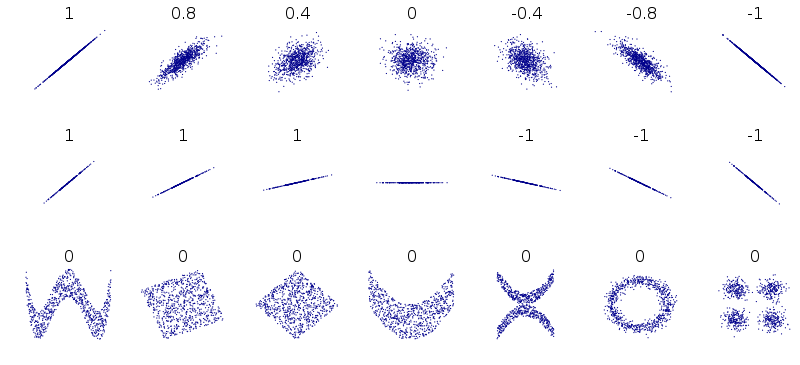Disclaimer: this figure (from wikipedia) shows correlation values associated with these datasets, but the limitations of correlation in capturing these patterns holds for linear regression as well.

## Polynomial regression: non-linear relationship between $$x$$ and $$y$$¶

Non-linear data can take all kinds of forms, though there are probably a few that are most common.

Let’s take a look at a simple example from our cars dataset:

sns.lmplot(data = mpg_clean, x = "horsepower", y = "mpg")

<seaborn.axisgrid.FacetGrid at 0x7f82186ecfd0>Does this data have a linear relationship between $$x$$ and $$y$$? Seems like it might be more complicated.

Enter: polynomial regression!

### Polynomial regression: overview¶

Polynomial regression is just like linear regression except that instead of fitting a linear function to the data, we fit higher degree polynomials.

Previously, our simple linear regression model assumed that our data $$(x_i, y_i)$$ could be described as:

$$y_i = \beta_0 + \beta_1 x_i + \epsilon_i$$

The OLS process estimates values for $$\beta_0$$ and $$\beta_1$$ that correspond to a straight line that minimizes $$\epsilon_i$$.

With polynomial regression, we extend this basic model to include functions of the form:

$$y_i = \beta_0 + \beta_1 x_i + \beta_2 x_i^2 + \epsilon_i$$ for degree 2 polynomial regression,

$$y_i = \beta_0 + \beta_1 x_i + \beta_2 x_i^2 + \beta_3 x_i^3 + \epsilon_i$$ for degree 3 polynomial regression,

$$y_i = \beta_0 + \beta_1 x_i + \beta_2 x_i^2 + \beta_3 x_i^3 + \ ... \ + \beta_n x_i^n + \epsilon_i$$ for degree n polynomial regression.

Even though this seems much more complex, polynomial regression uses the same Ordinary Least Squares (OLS) parameter estimation as simple regression. You can think of simple linear regression as a special case of polynomial regression.

This gives us immense flexibility to fit more complex functions to our data. Some of the data illustrated at the top of this section can only be modeled using more complex polynomials (see example below as well).

CAUTION: most of the time you don’t need polynomials to capture your data. Bad things happen when you use them for data that doesn’t have an underlying non-linear structure. More on this on Monday.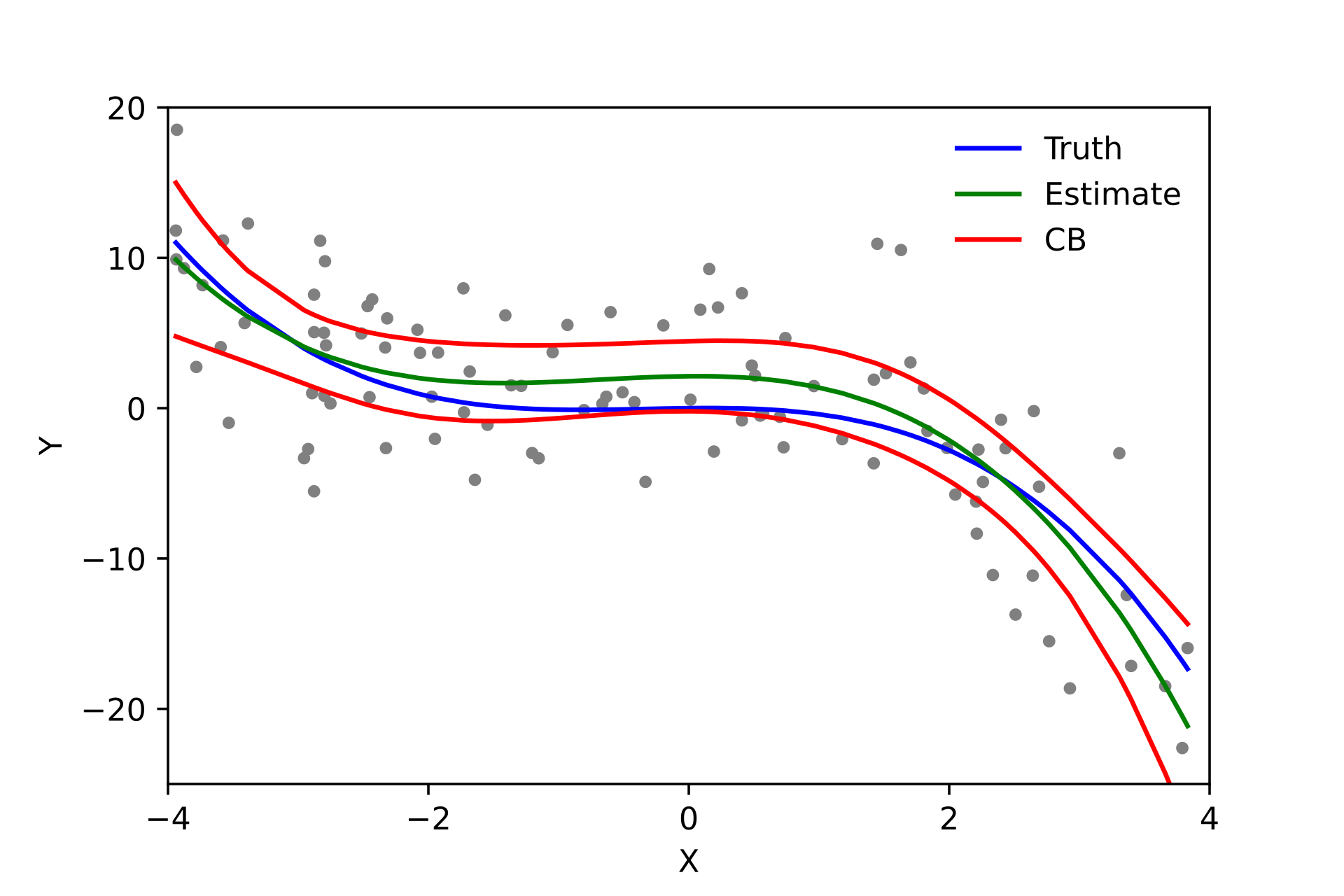### Polynomial regression in python¶

We can use the numpy polyfit library to fit 2nd and 3rd order polynomials to this data (Note: this is probably the simplest method, but there’s code to use the familiar scikit learn approach as well below).

# We can fit higher order polynomial functions to our data rather than just a linear function
deg1_fits = np.polyfit(mpg_clean.horsepower, mpg_clean.mpg, 1)
deg2_fits = np.polyfit(mpg_clean.horsepower, mpg_clean.mpg, 2)
deg3_fits = np.polyfit(mpg_clean.horsepower, mpg_clean.mpg, 3)

p1 = np.poly1d(deg1_fits)
p2 = np.poly1d(deg2_fits)
p3 = np.poly1d(deg3_fits)

# What do the functions fitted above predict for our data?

preds = mpg_clean.loc[:, ('horsepower', 'mpg')] #

preds['deg1_pred'] = p1(preds['horsepower'])
preds['deg2_pred'] = p2(preds['horsepower'])
preds['deg3_pred'] = p3(preds['horsepower'])

preds

preds_long = preds.melt(
id_vars = ['horsepower', 'mpg']
)
preds

horsepower mpg deg1_pred deg2_pred deg3_pred
0 130.0 18.0 19.416046 17.091508 17.153421
1 165.0 15.0 13.891480 13.480156 13.782683
2 150.0 18.0 16.259151 14.658717 14.890157
3 150.0 16.0 16.259151 14.658717 14.890157
4 140.0 17.0 17.837598 15.752059 15.904046
... ... ... ... ... ...
387 86.0 27.0 26.361214 25.908837 25.774667
388 52.0 44.0 31.727935 35.985609 36.424392
389 84.0 32.0 26.676903 26.422834 26.298564
390 79.0 28.0 27.466127 27.750895 27.662364
391 82.0 31.0 26.992593 26.946675 26.834765

392 rows × 5 columns

# First, our original data
sns.scatterplot(data = preds_long,
x = 'horsepower',
y = 'mpg',
color = 'm',
alpha = 0.1
)

# Now add in our lines
sns.lineplot(data = preds_long,
x = 'horsepower',
y = 'value',
hue = 'variable'
)

<AxesSubplot:xlabel='horsepower', ylabel='mpg'>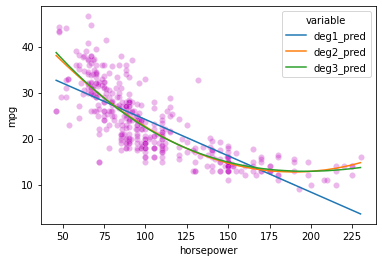Here’s the solution using scikit learn; it’s a bit more complicated, though it does let you keep using the LinearRegression class

from sklearn.preprocessing import PolynomialFeatures

x_vals = np.array(mpg_clean['horsepower']).reshape(len(mpg_clean['horsepower']), 1)
y_vals = np.array(mpg_clean['mpg'])
preds = mpg_clean.loc[:, ('horsepower', 'mpg')]

# Simple linear model
mod1 = LinearRegression().fit(x_vals, y_vals)

# 2nd order polynomial
poly2 = PolynomialFeatures(degree = 2, include_bias = False) # need include_bias = False
x2_features = poly2.fit_transform(x_vals)
mod2 = LinearRegression().fit(x2_features, y_vals)

# 3rd order polynomial
poly3 = PolynomialFeatures(degree = 3, include_bias = False)
x3_features = poly3.fit_transform(x_vals)
mod3 = LinearRegression().fit(x3_features, y_vals)

mod2.intercept_
# mod2.coef_
# mod3.coef_

56.90009970211295

# Add predictions for each model so we can view how it does
preds['deg1_pred'] = mod1.predict(x_vals)
preds['deg2_pred'] = mod2.predict(x2_features)
preds['deg3_pred'] = mod3.predict(x3_features)

preds

horsepower mpg deg1_pred deg2_pred deg3_pred
0 130.0 18.0 19.416046 17.091508 17.153421
1 165.0 15.0 13.891480 13.480156 13.782683
2 150.0 18.0 16.259151 14.658717 14.890157
3 150.0 16.0 16.259151 14.658717 14.890157
4 140.0 17.0 17.837598 15.752059 15.904046
... ... ... ... ... ...
387 86.0 27.0 26.361214 25.908837 25.774667
388 52.0 44.0 31.727935 35.985609 36.424392
389 84.0 32.0 26.676903 26.422834 26.298564
390 79.0 28.0 27.466127 27.750895 27.662364
391 82.0 31.0 26.992593 26.946675 26.834765

392 rows × 5 columns

preds_long = preds.melt(
id_vars = ['horsepower', 'mpg']
)
preds

# First, our original data
sns.scatterplot(data = preds_long,
x = 'horsepower',
y = 'mpg',
color = 'm',
alpha = 0.1
)

# Now add in our lines
sns.lineplot(data = preds_long,
x = 'horsepower',
y = 'value',
hue = 'variable'
)

<AxesSubplot:xlabel='horsepower', ylabel='mpg'>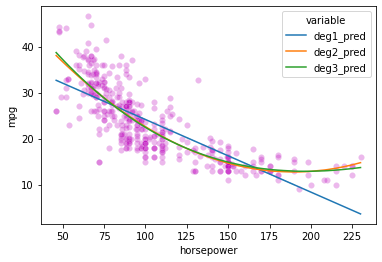# Let's check the R^2 values for these models to see what kind of improvement we get
# (more on this next week)


## Multiple regression: multiple predictors for $$y$$¶

Another basic scenario that arises when predicting a continuous variable (probably more commonly than polynomial regression) is having multiple predictors.

Let’s take a look at an intuitive example:

gap = pd.read_csv("https://raw.githubusercontent.com/UCSD-CSS-002/ucsd-css-002.github.io/master/datasets/gapminder.csv")

# Let's keep just some of the variables (note for pset!)
gap_subset = gap.loc[gap['year'] == 2007, ('country', 'year', 'lifeExp', 'pop', 'gdpPercap')]

gap_subset['logPop'] = np.log10(gap_subset['pop'])
gap_subset['logGdpPercap'] = np.log10(gap_subset['gdpPercap'])
gap_subset

country year lifeExp pop gdpPercap logPop logGdpPercap
11 Afghanistan 2007 43.828 31889923 974.580338 7.503653 2.988818
23 Albania 2007 76.423 3600523 5937.029526 6.556366 3.773569
35 Algeria 2007 72.301 33333216 6223.367465 7.522877 3.794025
47 Angola 2007 42.731 12420476 4797.231267 7.094138 3.680991
59 Argentina 2007 75.320 40301927 12779.379640 7.605326 4.106510
... ... ... ... ... ... ... ...
1655 Vietnam 2007 74.249 85262356 2441.576404 7.930757 3.387670
1667 West Bank and Gaza 2007 73.422 4018332 3025.349798 6.604046 3.480776
1679 Yemen, Rep. 2007 62.698 22211743 2280.769906 7.346583 3.358081
1691 Zambia 2007 42.384 11746035 1271.211593 7.069891 3.104218
1703 Zimbabwe 2007 43.487 12311143 469.709298 7.090298 2.671829

142 rows × 7 columns

In the last problem set, you generated a graph that predicted life expectancy as a function of income, with information about population and region available as well.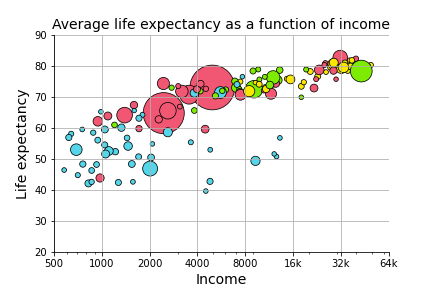The graph suggests that life expectancy is strongly predicted by income, while population may not play such an important role.

Let’s test that here!

What that amounts to asking is:

Can we predict life expectancy using both income and population better than we could only using one of those variables?

sns.scatterplot(data = gap_subset,
x = "logGdpPercap", # x1
y = "lifeExp",
color = "r"
)
plt.show()

sns.scatterplot(data = gap_subset,
x = "logPop", # x2
y = "lifeExp",
color = "b"
)
plt.show()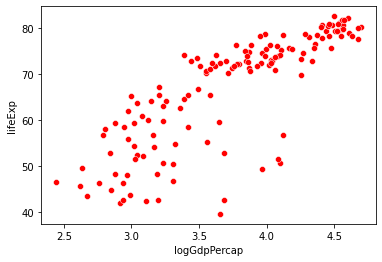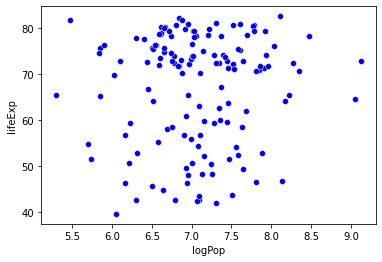### Multiple regression: overview¶

Multiple regression is like linear regression except that we assume our dependent variable $$y_i$$ is jointly predicted by multiple independent variables $$x_1$$, $$x_2$$, …, $$x_n$$, as in the example above.

As noted above, our simple linear regression model assumes that our data $$(x_i, y_i)$$ has the following form:

$$y_i = \beta_0 + \beta_1 x_i + \epsilon_i$$

With multiple regression, we now extend this model to include multiple predictors:

$$y_i = \beta_0 + \beta_1 x_{i,1} + \beta_2 x_{i,2} + \ ... \ + \beta_n x_{i,n} + \epsilon_i$$

In most cases, multiple regression once again uses the same Ordinary Least Squares (OLS) parameter estimation as simple regression. However, interpreting the parameter estimates is a little less straightforward.

How would we interpret $$\beta_0 = 1$$, $$\beta_1 = 2$$, $$\beta_2 = 3$$?

### Multiple regression in python¶

To run our multiple regression, we can use the scikit LinearRegression class with just a few modifications to our simple regression code.

I’ve also included the statsmodels code below as well so we can look at the statistics more closely!

# scikit learn approach
x_vals = np.array(gap_subset[['logGdpPercap', 'logPop']]) # Note: this syntax is important!
x_vals = x_vals.reshape(len(gap_subset), 2)
x_vals

y_vals = np.array(gap_subset['lifeExp'])
y_vals

mod = LinearRegression().fit(X = x_vals, y = y_vals)

mod.intercept_
mod.coef_

array([16.6811828 ,  1.86827925])

# How well does our regression do?
mod.score(X = x_vals, y = y_vals)

0.6649393884784984


Using the statsmodels regression class, we can view statistical tests on our parameter fits

multiple_reg = smf.ols('lifeExp ~ logGdpPercap + logPop', data = gap_subset).fit()

# View the results
multiple_reg.summary()

Dep. Variable: R-squared: lifeExp 0.665 OLS 0.660 Least Squares 137.9 Tue, 14 Jun 2022 9.91e-34 18:22:34 -477.07 142 960.1 139 969.0 2 nonrobust
coef std err t P>|t| [0.025 0.975] -8.6161 7.538 -1.143 0.255 -23.520 6.288 16.6812 1.008 16.555 0.000 14.689 18.673 1.8683 0.896 2.086 0.039 0.098 3.639
 Omnibus: Durbin-Watson: 34.155 2.17 0 54.987 -1.183 1.15e-12 4.923 104

Notes:
 Standard Errors assume that the covariance matrix of the errors is correctly specified.